## Coupled Circuits

Two circuits are coupled when they can exchange energy by conductive, electromagnetic or electrostatic means. This subject is (was) generally treated in circuits courses, and has a reputation for being rather confusing and difficult. Since it is hard to teach, and the instructors themselves may have little acquaintance with it, the subject has probably nearly vanished from modern courses, in spite of its importance. It is, indeed, possible to submit a circuit to a circuit analysis program, and let the program get the figures. There are, however, two difficulties with this procedure. First, you only get numbers, not understanding, and second, it is very easy to come up with an incorrect circuit specification. Before computers, students relied on substitution in formulas (even texts encouraged this procedure), achieving little understanding and, usually, making arithmetic errors. At least the computer does not make arithmetic errors.

This page shows how understanding can be had through experiment, phasor diagrams, and proceeding from first principles. Incidentally, one gets the required numbers as well.

Electromagnetic coupling takes the form of a winding in the secondary circuit (the one that receives energy) linking flux produced by a current in the primary circuit (the one that transmits energy). This is always a mutual relation, so that energy is conserved. With an air core, the flux is proportional to the current causing it, and mutual inductance M is the ratio of the voltage in the secondary to the rate of change of primary current with time, and the unit is the henry. This is exactly the same as (self-)inductance L, which is the ratio of voltage to rate of change of current. For sinusoidal currents, the impedance is jωM = ωM /_90° ohms. In every case, the relation between the current direction and the polarity of the voltage must be determined by considering the mode of winding and the relative location of the two windings. In the unusual case of mutual inductance between the parts of the same circuit, the value of M can be positive or negative, depending on how the windings are arranged. It is always best never to depend on any rules, but to write down Kirchhoff's equation for the circuit to express the relationships.

### Air-Core TransformerThe first example is of circuits coupled electromagnetically, by a mutual flux linking primary and secondary windings of an air-core transformer. The first necessity is, of course, to obtain the windings, which should be arranged so that the coupling between them can be varied. I used the cardboard tubes from rolls of paper towels and rolls of toilet paper. The toilet paper roll fit nicely over the paper towel roll, with space for the windings. I used #26 enameled wire for the windings. This is the smallest wire that I can control with any confidence. The wire was pushed through holes pricked in the cardboard at the ends of the windings, and secured with cellophane tape. As shown in the figure, one winding had about 50 turns, the other 75, but these are just suggestions, and there is no reason why they have to be different, except for sheer variety.

The inductance of the windings was measured by using a 100Ω resistor in series with the winding, and measuring the voltage across both, as well as across the resistor alone. A frequency of 500 kHz was used in all measurements. The voltage across the resistor gave the current, while the voltage across the inductance was the square root of the total voltage squared minus the resistor voltage squared. I found the longer winding to be 166 μH, and the shorter 108 μH, about what is expected from the empirical formula. The mutual inductance for the windings on top of each other was 111 μH (secondary voltage divided by primary current). This corresponds to a coefficient of coupling k = M/√L1L2 = 0.83, about the maximum possible.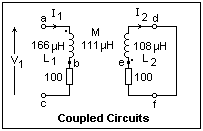The circuit to be studied is shown at the left. 100Ω resistors were used in both primary and secondary to make things interesting, and the secondary was shorted. Alternatively, the 100Ω resistor in the secondary can be thought of as the load, and the resistor in the primary as part of the source impedance. Notice the polarity marks on the windings. This means that an increasing I1 produces a positive voltage at the marked end of the secondary winding. This question of the polarity of the windings is one thing that makes coupled circuits interesting, and you simply have to figure it out from the way the coils are wound. The positive directions of the primary and secondary currents have been arbitrarily chosen in this case. This has an effect on the phasor diagram and on the measurements that must not be ignored.

Voltage ac (c grounded) is V1, while voltage bc gives I1. A positive voltage means a positive current. Voltage ef gives -I2. Use the oscilloscope to measure these three voltages, magnitude as well as phase relative to V1. I set V1 to 2.0 V, and found that I1 was 7.0 mA at an angle -40°. The measurement of the phase angle was quite rough and approximate. I2 was also 7.0 mA, at an angle of about 160° (taking the minus sign into account). We can now see how closely these results are predicted by circuit analysis.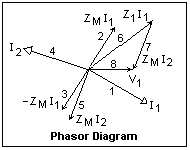The phasor diagram is shown at the right. The numbers are the order of drawing the phasors in the solution of the circuit. To draw a phasor diagram, you need a straightedge and scale, a protractor, two triangles for drawing parallel lines, and dividers for transferring distances. Each phasor should be carefully drawn to scale. If you can draw a phasor, you know how to calculate the corresponding quantity.

Start with the phasor representing the current I1. If it is unknown, simply assume 1 /_ 0°. The actual value can be found when you know the voltage V1. Since we have made a measurement, let's assume I1 = 7.0 /_-40°, and see if we come out with the applied voltage 2.0 /_ 0°. Now I1 induces a voltage -ZMI1 = 2.443 /_-90° V in the secondary. The minus sign comes from the fact that the polarity mark on the winding is opposite to the assumed positive direction of I2. Now you can draw phasor 2 and its negative, phasor 3. This voltage produces a current I2 in the impedance Z2 = 100 + j339 = 353 /_73.56° ohms. Hence, I2 = 6.92 /_-163.56°. Our measurement agrees well with this result. I12 induces a voltage -ZMI2 = 2.415 /_-73.56° in the primary (phasor 5), which adds to the voltage drop in the primary due to Z1 = 100 + j522 = 531 /_79.16° ohms, which is 3.717 /_79.16° (phasor 6). Now phasor 5 is moved to the tip of phasor 6 as phasor 7, and added to it to get V1. The result is 1.92 /_4° V, again close to the measured value. The phasor diagram can be measured to find these results, which are, of course, the same as we find by complex analysis. The two parallel calculations check each other.

Note that we have not required any solutions by determinants, or other error-prone methods, or any special formulas, and have obtained a pretty good idea of how the circuit behaves. The complex analysis is carried out using the rectangular-polar conversion facility of the pocket calculator, which makes it very easy and accurate. It all could be done purely graphically, or purely numerically, but this combination is by far the most convenient and accurate.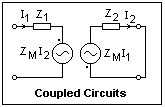A transformer is said to "reflect" an impedance in the secondary into the primary circuit. Consider the coupled circuits shown in the diagram at the left. The positive direction of the currents is chosen into the polarity mark on the generator representing the induced voltages, so that Kirchhoff's equations are Z1I1 + ZMI2 = V1, and ZMI1 + Z2I2 = 0. ZM is the mutual impedance jωM, Z1 includes the source impedance, and Z2 the secondary load. These equations may be solved for the equivalent primary impedance Ze = V1/I1 = Z1 - ZM2/Z2. The reflected impedance is then ω2M2/Z2. Note that a resistance is reflected as a resistance, a capacitance as an inductance, and an inductance as a capacitance.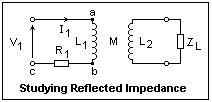You may well mistrust this formula and wonder how it applies in practice. The cure for this is to make some measurements and observe its consequences. A circuit for this is shown at the right. The idea is to connect various load impedances ZL and observe the effects on the primary voltage V1 and primary current I1. I used the coils from above, tightly coupled at k = 0.8, and R = 100Ω. Voltages ac and bc are displayed on two channels of the oscilloscope, the first giving V1, and the second I1. The frequency was 500 kHz.

With ZL = ∞ (open circuit), the ratio of V1 to I1 is Z1, the primary impedance, in my case about 100 + j516 Ω. The current lags by about 78° in the predominantly inductive circuit. Measuring the secondary voltage yields ωM = V2/I1. I used M = 111 μH, so that ωM = 349Ω. The inductance of the secondary is about 108 μH (from measurements made above, or by a special measurement), so that Z2 = j339 Ω.

When the secondary is shorted, ZL = 0, the reflected impedance is (ωM)2/j339 = 122000 / j339 = -j360. The effective primary impedance is now Ze = 100 + j516 - j360 = 100 + j156 = 185 /_57°. Actual measurement gave 188 /_54°--close enough considering the roughness of my measurements. This confirms the puzzling prediction that an inductance in the secondary is reflected as a capacitance in the primary. If we had measured the impedance, found the difference from the open-secondary case, and reflected this into the secondary (using the same formula), we would have obtained the secondary impedance Z2. Since we assumed that this was already known, we had a check on the theory.

I now connected a 120 μH inductor as ZL. This impedance of j377 added to the secondary impedance of j339 to make a total of j716, which reflects as -j170. The effective primary impedance is now 100 + j516 - j170 = 100 + j346 = 360 /_74°. Measurements of V1 and I1 yielded 359 /_74°, excellent agreement indeed. The close agreement is fortuitous, of course, but again shows that we are on the right track.

With an .001 μF capacitor for ZL, the surprising result was that the current I1 was nearly zero. In fact, the oscilloscope showed the second harmonic predominately. The effective impedance was very large. The load impedance was -j318, so the secondary impedance was j339 - j318 = j21. This was near-resonance, reflecting as -j5810 and dominating the effective primary impedance. If I could have seen the current, it would be leading by nearly 90°. This capacitive behavior can only happen near resonance in the secondary, when the secondary impedance is very low and inductive.

Using an .0033 μF capacitor as a load, the effective impedance was rather low, 125Ω, and the current led by 14° or so. Calculation gives ZL = -j96.4, Zs = j243, Zr = -j502, so Ze = 100 + j14 = 101 /_7.9°. The impedance is low, and the phase angle small, as expected, but there is not exact agreement. More care would be necessary to get better results. If .0043 μF is used instead, the current now lags, while the impedance remains low at 150Ω. Between these two values, we must have zero phase angle resonance, as the primary inductance is cancelled by the reflected capacitance (the secondary has a net inductance). Note that there are two resonance cases, one for secondary resonance, and one for primary resonance.

The predictions of the reflected-impedance formula appear to agree with measurements, and explain the curious phenomena. Note that the amount of the reflected impedance is proportional to (ωM)2, which changes quite rapidly as the degree of coupling changes. For small k, the effects are small, decreasing as k2. Of course, this is the reason I used a large k for the measurements.

### Double-Tuned Circuit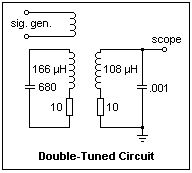We now look at the curious properties of coupled tuned circuits. The diagram at the left shows the two coils we made above, arranged to resonate at 500 kHz. I was fortunate that the 0.001 μF capacitor resonated the smaller coil, and a 680 pF ceramic capacitor resonated the larger one at the same frequency. In general, it will be convenient to use trimmer capacitors to set the resonant frequencies of the two coils equal. Resonance curves can be run to determine the Q of each resonant circuit. Calculated values are Q = 50 for the larger coil, Q = 34 for the smaller. The 10Ω resistors are necessary to bring the Q down to emphasize the effect we are seeking. It is quite important that the coupling between the coils can be varied. The circuit is excited inductively by a few turns of wire pushed in the end of the coils.

With tight coupling (k = 0.8) the resonant frequency will be pushed to considerably lower frequencies. What we are looking for is the double-peak response that appears at moderate coupling. Slide the coils apart to reduce the coupling, and look at the amplitude of the output as the frequency is scanned. With rather loose coupling, I was able to get a very distinct double peak. Since the coils are not identical, the response is not symmetrical. The smaller peak was at 460 kHz, the larger at 515 kHz, with a valley between them at 480 kHz. This is a very distinct effect, and cannot be missed. As the coupling is reduced, the response becomes single-peaked at critical coupling, and then decreases as the coupling is made even looser.

The broadening of the resonance is used in radio receivers to produce a sufficiently wide passband for the modulation, while retaining high Q selectivity. The bandwidth BW = kfo, where k is the coefficient of coupling and fo is the resonant frequency of each circuit.

The critical coupling coefficient is given by kc = 1/√Q1Q2, in terms of the Q's of the individual tuned circuits. Optimum coupling occurs for k = 1.5kc. In this case, the response is double-peaked, but does not dip much between the peaks and the response is close to what is ideally required. For tighter coupling, the bandwidth becomes wider, the peaks sharper and the dip more prominent.

The behavior of the circuit can be understood qualitatively on the basis of the reflected impedance. At resonance, the reflected impedance is resistive, and acts to lower the Q of the primary, and thereby to reduce the output. This is counteracted by the increased coupling, which increases the output. The lower Q gives a wider passband, as is noted. At frequencies lower than exact resonance, the reflected impedance is inductive, which adds to the inductance of the primary and resonates at a lower frequency, producing a peak in the output. At frequencies higher than exact resonance, the reflected impedance is capacitive, which cancels part of the inductance and causes the circuit to resonate at a higher frequency, producing the other peak.